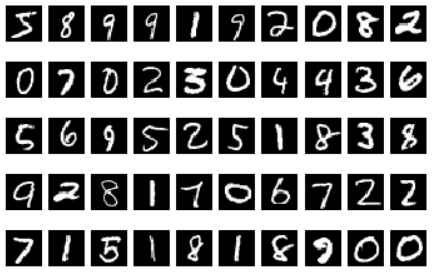# Softmax Regression

Original article was published on Artificial Intelligence on Medium## Build a Softmax Regression Model from Scratch in Python!

In my previous article, we learn about logistic regression which is used for binary classification. However, in real world application, there might be more than 2 classes to be classified, for example, digits classification. In this case, we call it multinomial logistic regression or also known as Softmax Regression.

## Derivation of Softmax Equation

Consider a classification problem which involved k number of classes.
Let x as the feature vector and y as the corresponding class, where y ∈ {1, 2, … , k}.

Now, we would like to model the probability of y given x, P(y|x), which is a vector of probabilities of y be either of the classes given the features: